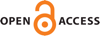Download this articleFor screen For printingRecent IssuesThe Journal About the Journal Editorial Board Editorial Interests Editorial Procedure Subscriptions Submission Guidelines Submission Page Policies for Authors Ethics Statement ISSN (electronic): 1364-0380 ISSN (print): 1465-3060 Author Index To Appear Other MSP JournalsDuality between Lagrangian and Legendrian invariants

### Tobias Ekholm and Yankı Lekili

Geometry & Topology 27 (2023) 2049–2179##### Abstract

Consider a pair $\left(X,L\right)$ of a Weinstein manifold $X$ with an exact Lagrangian submanifold $L$, with ideal contact boundary $\left(Y,\Lambda \right)$, where $Y$ is a contact manifold and $\Lambda \subset Y$ is a Legendrian submanifold. We introduce the Chekanov–Eliashberg DG–algebra, ${\mathrm{CE}}^{\ast }\left(\Lambda \right)$, with coefficients in chains of the based loop space of $\Lambda$, and study its relation to the Floer cohomology ${\mathrm{CF}}^{\ast }\left(L\right)$ of $L$. Using the augmentation induced by $L$, ${\mathrm{CE}}^{\ast }\left(\Lambda \right)$ can be expressed as the Adams cobar construction $\mathrm{\Omega }$ applied to a Legendrian coalgebra, ${\mathrm{LC}}_{\ast }\left(\Lambda \right)$. We define a twisting cochain $\mathfrak{𝔱}:{\mathrm{LC}}_{\ast }\left(\Lambda \right)\to B{\left({\mathrm{CF}}^{\ast }\left(L\right)\right)}^{#}$ via holomorphic curve counts, where $B$ denotes the bar construction and $#$ the graded linear dual. We show under simple-connectedness assumptions that the corresponding Koszul complex is acyclic, which then implies that ${\mathrm{CE}}^{\ast }\left(\Lambda \right)$ and ${\mathrm{CF}}^{\ast }\left(L\right)$ are Koszul dual. In particular, $\mathfrak{𝔱}$ induces a quasi-isomorphism between ${\mathrm{CE}}^{\ast }\left(\Lambda \right)$ and $\mathrm{\Omega }{\mathrm{CF}}_{\ast }\left(L\right)$, the cobar of the Floer homology of $L$.

This generalizes the classical Koszul duality result between ${C}^{\ast }\left(L\right)$ and ${C}_{-\ast }\left(\mathrm{\Omega }L\right)$ for $L$ a simply connected manifold, where $\mathrm{\Omega }L$ is the based loop space of $L$, and provides the geometric ingredient explaining the computations given by Etgü and Lekili (2017) in the case when $X$ is a plumbing of cotangent bundles of $2$–spheres (where an additional weight grading ensured Koszulity of $\mathfrak{𝔱}$).

We use the duality result to show that under certain connectivity and local-finiteness assumptions, ${\mathrm{CE}}^{\ast }\left(\Lambda \right)$ is quasi-isomorphic to ${C}_{-\ast }\left(\mathrm{\Omega }L\right)$ for any Lagrangian filling $L$ of $\Lambda$.

Our constructions have interpretations in terms of wrapped Floer cohomology after versions of Lagrangian handle attachments. In particular, we outline a proof that ${\mathrm{CE}}^{\ast }\left(\Lambda \right)$ is quasi-isomorphic to the wrapped Floer cohomology of a fiber disk $C$ in the Weinstein domain obtained by attaching ${T}^{\ast }\left(\Lambda ×\left[0,\infty \right)\right)$ to $X$ along $\Lambda$ (or, in the terminology of Sylvan (2019), the wrapped Floer cohomology of $C$ in $X$ with wrapping stopped by $\Lambda$). Along the way, we give a definition of wrapped Floer cohomology via holomorphic buildings that avoids the use of Hamiltonian perturbations, which might be of independent interest.

##### Keywords
Lagrangian, Legendrian, Floer cohomology, Chekanov–Eliashberg dg–algebra
Primary: 57R17
##### Publication